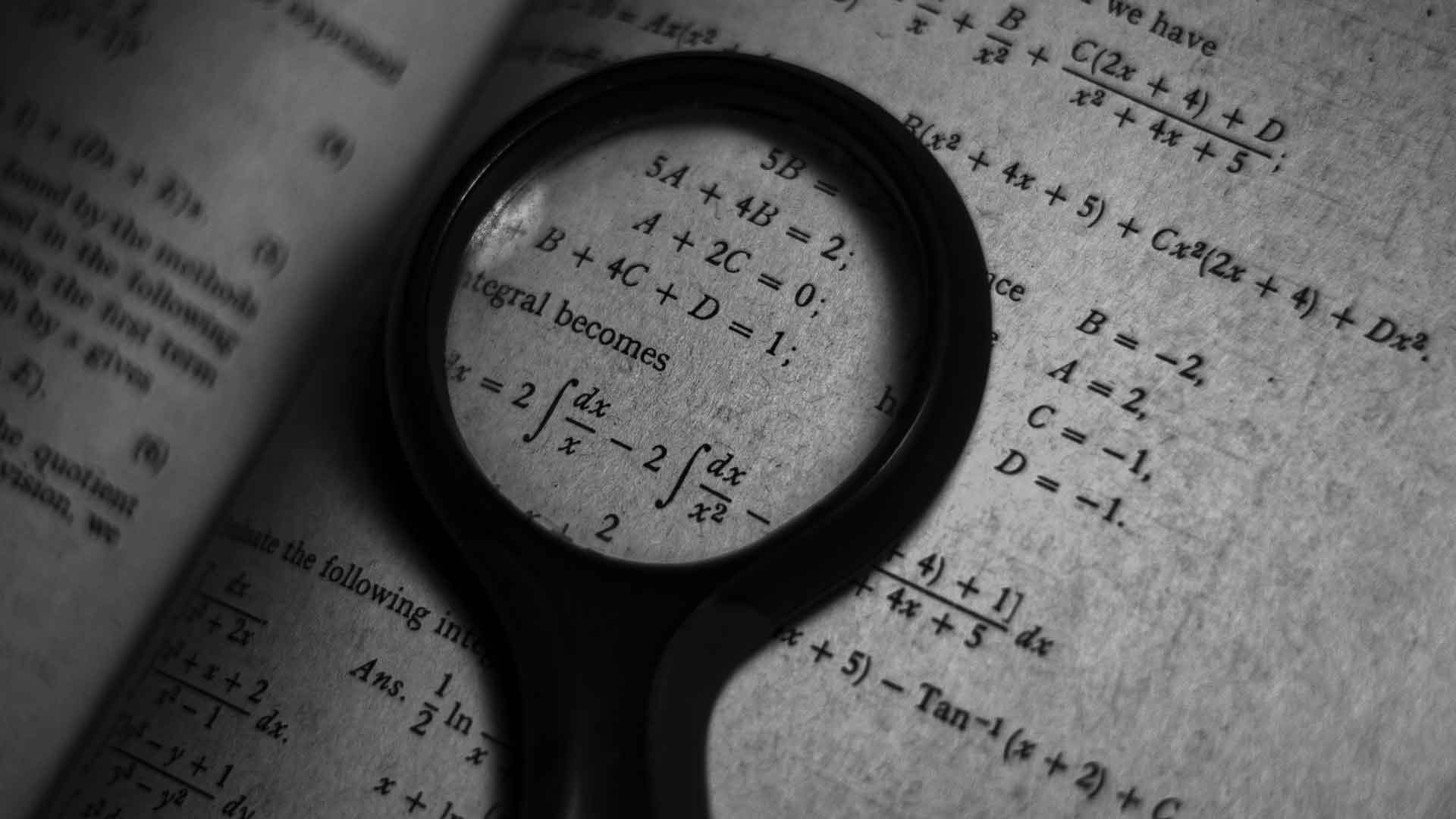Fact checked# What are Actuarial Mathematics?

Brief and Straightforward Guide: What are Actuarial Mathematics? (with pictures)
Mathematics3 minutesActuarial science refers to the unique blend of several different fields of study; it serves the purpose of providing quantifiable guidelines for business decisions involving risk evaluation. The mathematics required by this science is a complex blend of calculus, statistics, financial math, and numerical modeling. Actuarial mathematics is used to support solutions to a number of different problems in business and government.

Calculus is required in actuarial mathematics because this topic of mathematics is concerned with change. Many problems solved by actuaries involve change over time. Examples are how a variable changes with age of the study population or mechanical reliability changes with operating hours. Calculus provides the functions to describe systems and the means to evaluate limits of those systems. Integral calculus sums a variable’s changes over time, and differential calculus looks at changes per unit time.

The actions of people and their life events are studied as part of actuarial mathematics using statistics and probability to predict future outcomes. The science of statistics attempts to predict responses from past behaviors. It distinguishes between random and nonrandom events and tries to remove randomness from a system to allow predictability.

The time value of money is the basis for many financial math problems. Recognizing that this asset fluctuates in value over time complicates the decision-making process. Not only does actuarial mathematics address varying economic scenarios such as increasing or decreasing interest rates, it also must incorporate the functions of calculus into the analysis. Changing financial environments are piled on top of changes in the main variable over time.

Numerical modeling offers some relief to the field of actuarial mathematics. By breaking down the problem into minute sub-problems and using approximations of values at the boundaries of the sub-problems, simple equations may be used. These techniques still need to model the actual method by which change occurs to the extent possible. Often their use is limited to part of a problem. Numerical modeling of a disease mechanism may produce a theoretical input population to an algorithm that is then solved more rigorously.

Computer science is often studied as part of actuaries’ model curriculum. The complexity of the problems attempted or the use of numerical approximations usually mandates that a computer's ability to calculate equations repeatedly be applied. Actuary science was greatly enhanced with the development of the small computer.

Many industries benefit from actuarial mathematics. Life insurance tables and financial risks of investments are common uses. Risk evaluations of major engineering projects can help avoid catastrophic results financially and in the lives of people living near the project. Governments use actuarial mathematics in evaluating the probabilities and effects of simulated foreign policy decisions. War games may also be used in the teaching of actuarial mathematics.

Do you need a math tutor to work with you one-on-one? Contact our academic advisor today to get matched with an online math tutor!### Get started with a custom-matched tutor for your child.